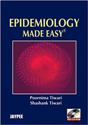# Epidemiology: Tests of SignificanceCommonly used tests of significance are

For qualitative data: (comparing two proportions)

• Standard error of difference between two proportions
• Chi – square test

For quantitative data: (comparing two means)

• Unpaired – t test (the t – test)
• ‘Z’ – test
• Paired t – test

Chi – square test
• Applied on qualitative data
• Equally applicable on small and large samples
• Can be applied if there are more than 2 categories

Std. error of diff between proportions test
• Applied on qualitative data
• Applicable on larger samples only (≥30 participants)
• Can compare the proportions between two groups only

Unpaired or student’s t – test

• Calculate the observed difference between the two means
• Calculate the standard error of difference between the means of the two groups
• Calculate the t – value as:
t= (observed difference between the two means)/(standard error of difference between the means)
• Determine the degrees of freedom as:
D.f. = n1 + n2 -2
Where n1= number of subjects in the first group
And n2 = number of subjects in the second group
Refer to t – distribution table
• Look against the calculated d.f. and observe the t value under p=0.05
• If the calculated value exceeds this value of t, the p is

The Z – test
• Applicable only in larger samples
• Z is calculated instead of t
And:
 If Z=1.56, p value is 0.10
 If Z=1.96, p value is 0.05
 If Z=2.3, p value is 0.02
 If Z=2.58, p value is 0.01
Higher the value of Z, lower is the p – value

Paired t – test:

Used when:
• Single sample but each subject gives two values (paired values) of the same variable
• Quantitative data
• Random sample
Examples of where paired t – test is applicable:
 Value before and after treatment e.g. systolic BP of each subject before taking treatment and the systolic BP of the same subject after treatment
 Comparing effects of two different drugs on the same individual for example blood sugar level after 2 hours of drug A and the same after drug B
 Checking the accuracy of a new measuring instrument against a standard one, for example Hb estimation by an new method against the same by colorimeter
For more details on the tests tests of significance
References:
Tiwari P. Epidemiology Made Easy. New Delhi: Jaypee Brothers; 2003
Gordis, L. (2014). Epidemiology (Fifth edition.). Philadelphia, PA: Elsevier Saunders.
Bonita, R., Beaglehole, R., Kjellström, T., & World Health Organization. (2006). Basic epidemiology. Geneva: World Health Organization.
Schneider, Dona, Lilienfeld, David E (Eds.), 4th ed. Lilienfeld’s Foundations of Epidemiology. New York: Oxford University Press; 2015
K. Park. Principles of Epidemiology and Epidemiologic Methods. In Park’s Textbook of Preventive and Social Medicine. 24th Ed. Jabalpur: Banarasidas Bhanot, 2017: pg 58 – 145

Steps in Investigation of an Epidemic: http://www.ihatepsm.com/blog/epidemiology-steps-investigation-epidemic
Tests of Significance: http://www.ihatepsm.com/blog/epidemiology-tests-significance
Monitoring and Evaluation: http://www.ihatepsm.com/blog/epidemiology-monitoring-and-evaluation